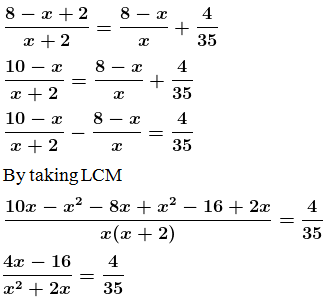Guru

# The sum of the numerator and denominator of a certain positive fraction is 8. If 2 is added to both the numerator and denominator, the fraction is increased by 4/35. Find the fraction

• 1

This question has been taken from Book:- ML aggarwal, Avichal publication, class10th, quadratic equation in one variable, chapter 5, exercise 5.5
This is an important ques and asked in exam

The sum of the numerator and denominator of a certain positive fraction is 8.

If 2 is added to both the numerator and denominator,

the fraction is increased by 4/35. Find the fraction
Question no10. ,ML Aggarwal, chapter 5, exercise 5.5, quadratic equation in one variable, ICSE board,

Share

1. Solution:

Let the denominator be ‘x’

So the numerator will be ‘8-x’

The obtained fraction is (8-x)/x

So according to the question,By cross multiplying,

35(4x – 16) = 4(x+ 2x)

140x – 560 = 4x2 + 8x

4x2 + 8x – 140x + 560 = 0

4x2 – 132x + 560 = 0

Divide by 4, we get

x2 – 33x + 140 = 0

let us factorize,

x2 – 28x – 5x + 140 = 0

x(x – 28) – 5 (x – 28) = 0

(x – 28) (x – 5) = 0

So,

(x – 28) = 0 or (x – 5) = 0

x = 28 or x = 5

So the value of x = 5 [since, x = 28 is not possible as sum of numerator and denominator is 8]

When substitute the value of x = 5 in the fraction (8-x)/x, we get

(8 – 5)/5 = 3/5

∴ The required fraction is = 3/5

• 1